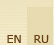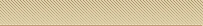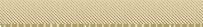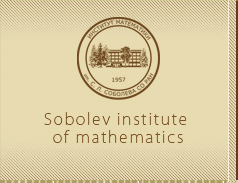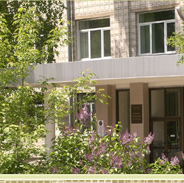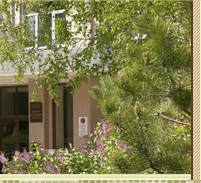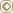MainWeb-postSite mapS. L. S o b o l e vPublicationsS e r g e i  L'v o v i c h  S o b o l e v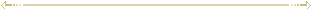Publications  (1972-1976) The second International congress on mathematical education held in Ecceter, England  (Russian) // Mat. v shkole. — No. 6. — P. 84-87. — With G. G. Maslova. Vladimir Iosifovich Kondrashov (Obituary). (Russian) // Uspekhi Mat. Nauk. — 1972. — Vol. 27, No. 2. — P. 149-155. — With L. D. Kudryavtsev et al. Zbl. 231.01007 MR0384432 (52 #5309) Vladimir Iosifovich Kondrashov: (Obituary) (English) // Russ. Math. Surveys. — 1973. — Vol. 27, No. 2. — P. 83-90. — With L. D. Kudryavtsev et al. Zbl. 249.01015 Some features of mathematical education in the USSR (Russian) // International congress of mathematicians in Nice, 1970. — Moscow, 1972. — P. 290-300. To be equal with the epoch (Russian) // Lit. gas. — 1972. — May 1. — No. 18. When creative inspiration comes (Russian) // Lit. gas. — 1972. — June 21. — No. 25. What does grade «five» mean? (Russian) // Izvestiya. — 1972. — February 10. On optimal cubature formulas in a finite region (Russian) // Theory of cubature formulas and applications of functional analysisto some problems of mathematical physics: Materialy shk. konf. — Ulan-Ude, 1973. — Novosibirsk, 1973. — P. 5-21. Introduction to the report by Р. Thoma on the second International congress on mathematical education «Modern mathematics - does it exist?» (Russian) // Mat. v shkole. — 1973. — No. 1. — P. 89. Mathematical education in the USSR: [Report on the 2 International congress on mathematical education, 1972.] (Russian) // Mat. v shkole. — 1973. — No. 1. — P. 4-10. Ed.: Theory of cubature formulas and applications of functional analysis to some problems of mathematical physics (Russian) // Mater. shk. konf., Ulan-Ude, 1973. — Novosibirsk: Nauka, 1973. — 251 pp. Mathematics’ muse (Russian) // Nedelya. — 1973. — No. 29. — P. 8-9. I prefer active rest (Russian) // Za nauku v Sibiri. — 1973. — March 21. — No. 12. Introduction to the theory of cubature formulae (Russian) — Moscow: Nauka, 1974. — 808 pp. Zbl. 294.65013 MR0478560 (57 #18037) [Short variant]: Cubature Formulas and Modern Analysis: An Introduction (English) — Montreux: Gordon and Breach Sci. Publ., 1992. — 379 pp. Zajd Ismailovich Khalilov: (Nachruf) (Russian) // Uspekhi Mat. Nauk. — 1974. — Vol. 29, No. 5. — P. 211-214. — With A. V. Bitsadze et al. Zbl. 229.01014 Zaid Ismailovich Khalilov: (Obituary) (Russian) // Uspekhi Mat. Nauk. — 1974. — Vol. 29, No. 5. — P. 211-214. — With A. V. Bitsadze et al. MR0386942 (52 #7791) Zajd Ismajlovich Khalilov: (Obituary) (English) // Russ. Math. Surveys. — 1974. — Vol. 29, No. 5. — P. 209-212. — With A. V. Bitsadze et al. Zbl. 314.01022 Ed.: Godunov  S. K., Zolotareva  E. V. Collection of problems on mathematical physics equations (Russian) — Novosibirsk, Nauka, 1974. — 74 pp. Some words about mathematics (Russian) // Za nauku v Sibiri. — 1974. — February 20. — No. 8. Our principal task is to find new methods and to develop horizons of our science (Russian) // Za nauku v Sibiri. — 1974. — November 27. — No. 46. Mathematics in the Institutes of higher education (Russian) // Mathemat. obrazovanie segodnya. — Moscow, 1974. — P. 13-24. Ol’ga Arsen’evna Oleinik (Russian) // Vestnik Moskov. Univ. Ser. I Mat. Meh. — 1975. — No. 4. — P. 119-122. — With P. S. Aleksandrov and A. N. Kolmogorov. MR0381908 (52 #2797) Convergence of cubature formulas on infinitely differentiable functions (Russian) // Dokl. Akad. Nauk SSSR. — 1975. — Vol. 223, No. 4. — P. 793-796. MR0408216 (53 #11981) Convergence of cubature formulas on infinitely differentiable functions (English) // Soviet Math. Dokl. — 1975. — Vol. 16. — P. 991-995. Zbl. 341.41025 Les formules optimales pour l’integration des fonctions de plusieurs variables // Metodi Valutativi Nella Fisica-Matematica: Conv. Intern., 1972, Roma. — Roma, 1975. — P. 423-441. Ed.: Theory of cubature formulas and applications of functional analysis to some problems of mathematical physics (Russian) // Mater. shk. konf., Tashkent, 1974. — Novosibirsk: Nauka, 1975. — 256 pp. Ol’ga Arsen’evna Oleinik (Russian) // Mat. v shkole. — 1976. — No. 2. — P. 81-83. Ivan Georgievich Petrovskii (Russian) // Problems in mechanics and mathematical phizics: Dedicated to the memory of I.G. Petrovskii — M., Nauka. 1976. — P. 1-6. — With A. Ju. Ishlinskii and O. A. Oleinik. MR0472419 (57 #12120) Convergence of cubature formulas on various classes of periodic functions (Russian) // Trudy Sem. S. L. Soboleva. — Novosibirsk, 1976. — No. 1. — P. 122-140. MR0568063 (81g:65041) Convergence of cubature formulas on elements L2m (Russian) // Dokl. Akad. Nauk SSSR. — 1976. — Vol. 228, No. 1. — P. 45-47. MR0411135 (53 #14874) Convergence of cubature formulas on the elements of L2m (English) // Soviet Math. Dokl. — 1976. — Vol. 17. — P. 660-663. Zbl 352.41031 Ed.: Problems in mechanics and mathematical phizics: Dedicated to the memory of I.G. Petrovskii (Russian) — Moscow: Nauka, 1976. — 298 pp. MR0444361 (56 #2715) Friendship solidifies strength: on the occasion of signing the Agreement between the USSR and Czechoslovakia (Russian) // Sov. Sibir’. — 1976. — December 12. Our faithful companions: [About literature] (Russian) // Lit. gazeta. — 1976. — March 10. — No. 10. [On mathematics and education] (Russian) // Za nauku v Sibiri. — 1976. — August 5. — No. 31.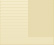©Sobolev Institute of Mathematics, 2009      Siberian Branch of the Russian Academy of Sciences4 Acad. Koptyug avenue, 630090 Novosibirsk Russia Tel.: (383) 333-28-92 Fax: (383) 333-25-98 E-mail: